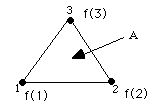**II. Refinement or Derefinement of Faces:** Each face is tested separately for refinement or derefinement. For the tetrahedral face defined by nodes 1, 2, and 3, we havewhere A is the area of the face. Criteria: 1) Junction: Refine if any of the faces field values straddle c. Tag for refinement if f(1) c and f(2), f(3) < c or f(2) c and f(1), f(3) < c or f(3) c and f(1), f(2) < c or all of the above with and < interchanged. example: For c = 0, refine if f changes sign between any of the three nodes. 2) Constant: Refine if any of the face field values exceed c. Tag for refinement if f(1) 2 or f(2) c or f(3) c. 3) Maxsize: Refine if the face area exceeds c. Tag if A c. 4) Aspect Ratio: Refine if the faces aspect ratio is less than c. The aspect ratio (AR) is defined as the ratio of the radius of the inscribed circle of the triangular face to the radius of the circumscribed circle. We renormalize this ratio of multiplying by 2 so that the ratio equals one for an equilateral triangle. AR = 2 RIN/ROUT where RIN = radius of inscribed circle ROUT = radius of circumscribed circle AR is never greater than one. Tag if AR < c. Generally the smaller AR is the more elongated the face is. 5) Lambda Refine: Refine if lamda/dx